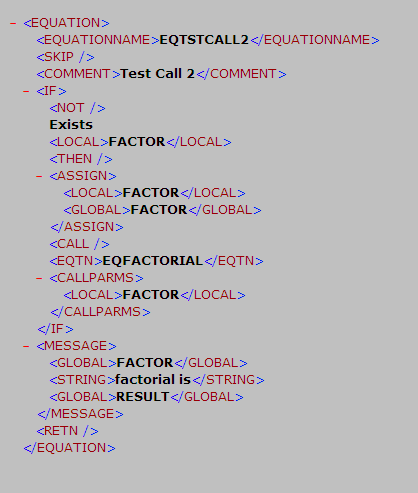Viewing Equations as Algebraic Expressions

This section discusses how to view equations as algebraic expressions.

You can view any equation that you are authorized to read on the Review Equations component (EQUATION_VIEW).

Page Name

Definition Name

Usage

Review Equations

SCC_EQUATION_VIEW

• select Set Up SACR, then select Common Definitions, then select Equation Engine, then select Review Equations

• Click Equation Detail on the Related Item Type Group page, Packaging Plan page, FM Target page, IM Target page, Equity Rule page, Packaging Limits page, or the Packaging Rules 1 page to review an equation in read-only mode.

View equations as algebraic equations.

Access the Review Equations page (select Set Up SACR, then select Common Definitions, then select Equation Engine, then select Review Equations).

Image: Review Equations page

This example illustrates the fields and controls on the Review Equations page. You can find definitions for the fields and controls later on this page.This page displays the equation name and an approximate algebraic representation of the equation. You cannot edit the equation in this page. Use the scroll bar to view the entire equation.

Note: This summary is a representation of the equation that you created on the Equation Editor page. The Equation Editor page determines how your equation functions.